2.3 The Level Shift

The Frank-Condon theory provides a theoretically profound description of vibronic transitions and has a wide range of applications in quantum chemistry, such as the calculation of the absorption and fluorescence spectra. In the following, this theory will be employed for the case of charge trapping into oxide defects and will lead to a shift of the defect levels. This level shift can be best explained using a configuration coordinate diagram of a defect (depicted in Fig. 2.3). The neutral defect is represented by the curve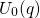, which can, for instance, be identified with the formation energy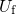of the defect1. In equilibrium, the neutral defect takes on its minimum configuration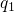labeled with A in Fig. 2.3. If the defect is charged positively, one electron has to be removed from the defect and placed within the substrate valence band, for instance at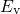. As a consequence, the electron energymust be added to the defect energy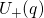for a correct comparison with. But one should consider that vibronic transitions can occur with each conduction or valence band state in the substrate, including the energies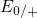and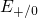. As mentioned before, the transition rates for charge trapping can be calculated using the Franck-Condon theory. The corresponding equation (2.21) depends most strongly on the factor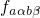, which is determined by the overlap of the nuclei wavefunctions. This factor reaches the largest values for vibrational transitions, whose energies lie close the intersection point (IP) of the adiabatic potentials. In the classical limit, it is even peaked at the energy of this intersection point, at which the vibronic transition is assumed to occur. During a hole capture process, the neutral defect is initially in its equilibrium configuration(state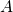in Fig. 2.3). There, the defect has an energy of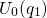, which only intersects with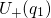when the emitted electron is placed at the energy levelin the substrate valence band. It is emphasized here that the defect is assumed not to overcome the barrier over the intersection point IP (path C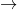IPA in Fig. 2.3) in the concept of the level shift. During the tunneling transition of the electron, the defect is not capable of adopting the new configuration of the new charge state according to the Franck-Condon principle. Afterwards, it is in the state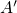and relaxes to the equilibrium configuration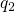(state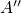). During this process, the defect dissipates the relaxation energy of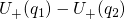to the heat bath. In the case of an electron capture process, the defect is initially in its equilibrium configurationlabeled with B in Fig. 2.3. A vibronic transition is only allowed, if a substrate electron is excited to the energy(from state A” to B) by the heat bath. Then the defect plus the electron have an energy of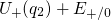(state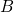in Fig. 2.3), which coincides with the energy of the neutral defect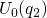. Analogously to the hole capture process, the defect configurationis preserved during the tunneling transition (state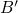). Afterwards the defect undergoes a structural relaxation to state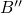thereby dissipating an energy of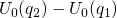to the heat bath. The aforementioned electron levelsandare usually referred to as switching levels and can be defined as the difference of adiabatic potential energies: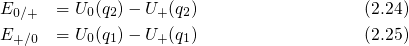There is a the shift between energy levels for electron () and hole () capture. This difference is ascribed to the fact that the defects have different equilibrium configurationsandfor different charge states of the defect. As a consequence, an ‘elastic’ vibronic transition can only occur at different energies in the configuration coordinate diagram. This requires that the exchanged electron has different energiesandfor electron and hole capture — a fact that has motivated the term ‘level shift’. It is important to note at this point that the above considerations are not restricted to defect transitions between positive and neutral charge states and can in principle be extended to all possible combinations of charge states.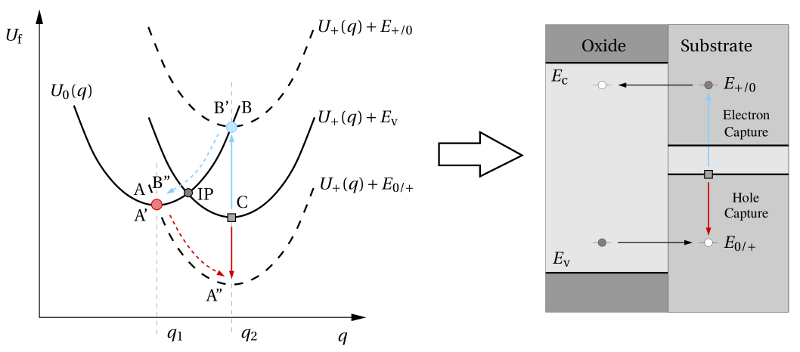Figure 2.3: The configuration coordinate diagram of a defect in its positive and neutral charge state (left) and the corresponding trap levels in the band diagram (right). The solid curves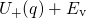andrepresents the adiabatic potentials of the positive and neutral defect system, respectively. In the former case, the tunneling electron is moved to the substrate where it sits at the valence band edge. When the electron has a different energy, for instanceor, the corresponding adiabatic potential is shifted on the energy scale and depicted by the dashed lines. The red and the blue filled circle indicates the points, where the hole and electron trapping process can occur. These points only represent the temporary states, which are realized right after the tunneling process. The band diagram on the right-hand side includes the defect levels extracted from the configuration coordinate diagram (left). The resulting electron () and hole capture () level can be separated by several electron Volts, where only one of these two levels can be active at a time. Interestingly, the energy levelmust always lie abovedue to the positive curvature of the adiabatic potentials.

In conclusion, the conventional concept of fixed trap levels must be questioned if a defect has distinct equilibrium configurations for two charge states. Instead, the trap/defect levels depend on their charge state, which can have a considerable impact on the trapping dynamics. Although this concept has not been applied to charge trapping so far, it can give an explanation for trap-assisted tunneling through dielectrics .